## 在编辑器中检查变量作用域

### 使用自动函数和变量高亮显示功能

• 在您将光标放在函数或变量名称内时以天蓝色高亮显示特定函数或局部变量的所有实例。例如：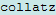• 无论光标位置在哪儿，都以水鸭蓝显示带有共享作用域的变量。例如：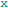### 使用自动函数和变量高亮显示功能的示例

```function rowTotals = rowsum % Add the values in each row and % store them in a new array x = ones(2,10); [n, m] = size(x); rowTotals = zeros(1,n); for i = 1:n rowTotals(i) = addToSum; end function colsum = addToSum colsum = 0; thisrow = x(i,:); for i = 1:m colsum = colsum + thisrow(i); end end end```

```ans = 10 10```

MATLAB 会转而显示以下结果：

```ans = 0 0 0 0 0 0 0 0 0 10```

1. 主页选项卡上的环境部分中，点击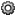并选择 MATLAB > 颜色 > 编程工具。确保选择自动突出显示具有共享范围的变量

2. `rowsum` 代码复制到编辑器中。

请注意变量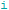以水鸭蓝显示，这表示 `i` 不是局部变量。`rowTotals` 函数和 `addToSum` 函数都设置和使用变量 `i`

第 6 行的变量 `n` 显示为黑色，表示它未跨越多个函数。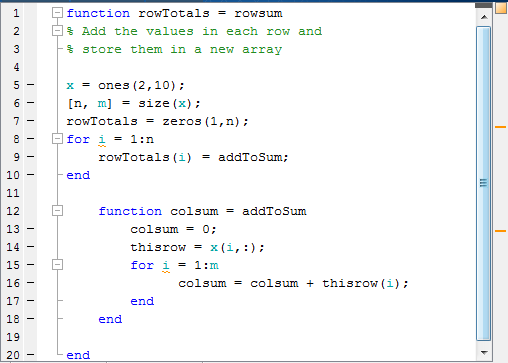3. 将鼠标指针悬停在变量 `i` 的实例上方。

随即出现工具提示：变量“i”的作用域跨越多个函数。

4. 点击工具提示链接以了解其作用域跨越多个函数的变量相关信息。

5. 点击 `i` 的实例。

`i` 的每次引用都会用天蓝色高亮显示，标记显示在编辑器右侧的指示标记条中。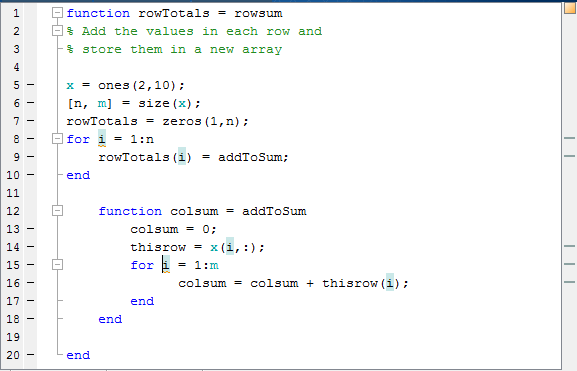6. 将鼠标光标悬停在某个指示标记条的标记上方。

随即出现工具提示，其中显示函数或变量名称以及用标记表示的代码行。

7. 点击标记以导航至在该标记的工具提示中指定的行。

如果您的文件包含许多代码，您在编辑器中不能一次查看完，上述做法非常有用。